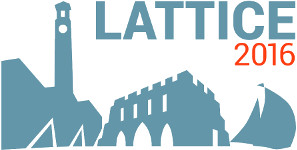#The 34th International Symposium on Lattice Field Theory (Lattice 2016)

Jul 24 – 30, 2016
Highfield Campus, University of Southampton
Europe/London timezone

## Leptonic decay-constant ratio $f_K/f_\pi$ from clover-improved $N_f=2+1$ QCD

Jul 28, 2016, 2:40 PM
20m
Building 67 Room 1003 (Highfield Campus, University of Southampton)

### Building 67 Room 1003

#### Highfield Campus, University of Southampton

Highfield Campus, Southampton SO17 1BJ, UK
Talk Weak Decays and Matrix Elements

### Speaker

Dr Enno E. Scholz (University of Regensburg)

### Description

The leptonic decay-constant ratio $f_K/f_\pi$ is calculated from lattice-QCD simulations using $N_f=2+1$ dynamical fermion flavors in the clover-improved formulation and 2-HEX smearing. The simulations were performed at a range of mass-degenerate light quarks including the physical point and at various lattice couplings and volumes, allowing to quantify all relevant sources of systematic uncertainties for our final number of the decay-constant ratio. Utilizing input from ChPT, we also quote the charged decay-constant ratio $f_{K^\pm}/f_{\pi^\pm}$. With further input from super-allowed nuclear $\beta$-decays, eventually we obtain an estimate for the CKM-matrix element $V_{\rm us}$.

### Primary author

Dr Enno E. Scholz (University of Regensburg)

### Co-author

Dr Stephan Durr (University of Wuppertal)

 Slides